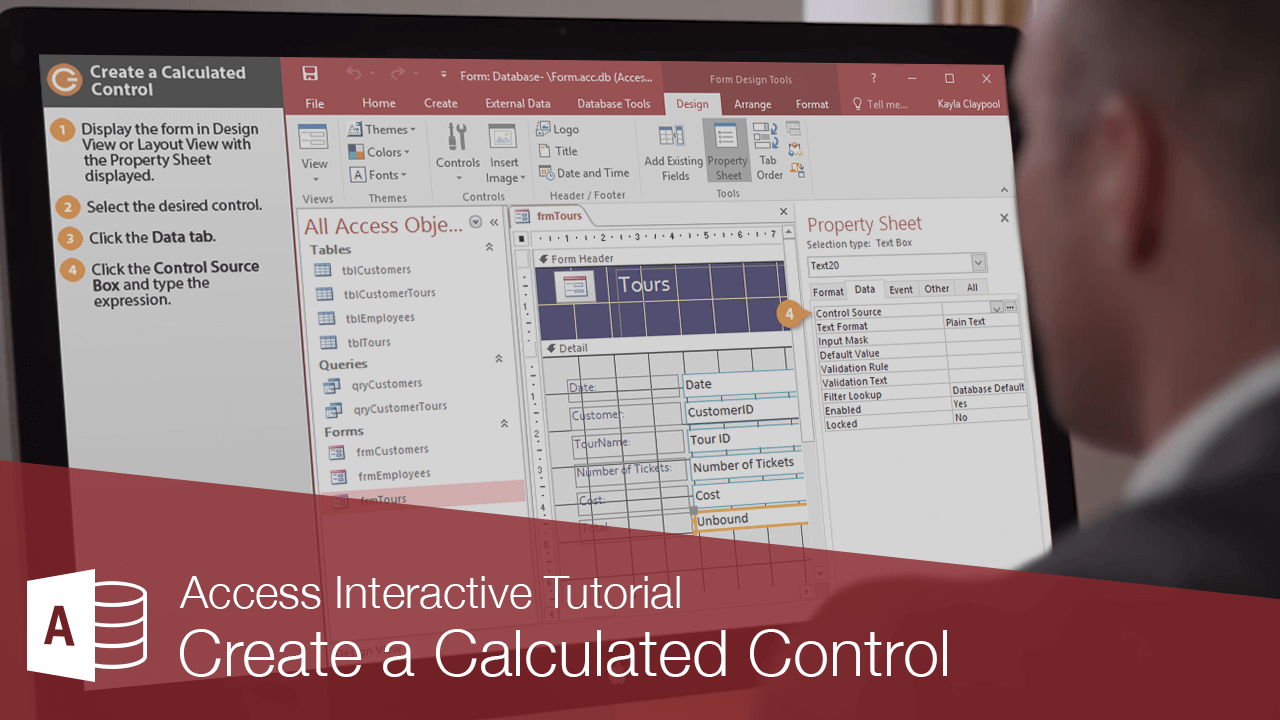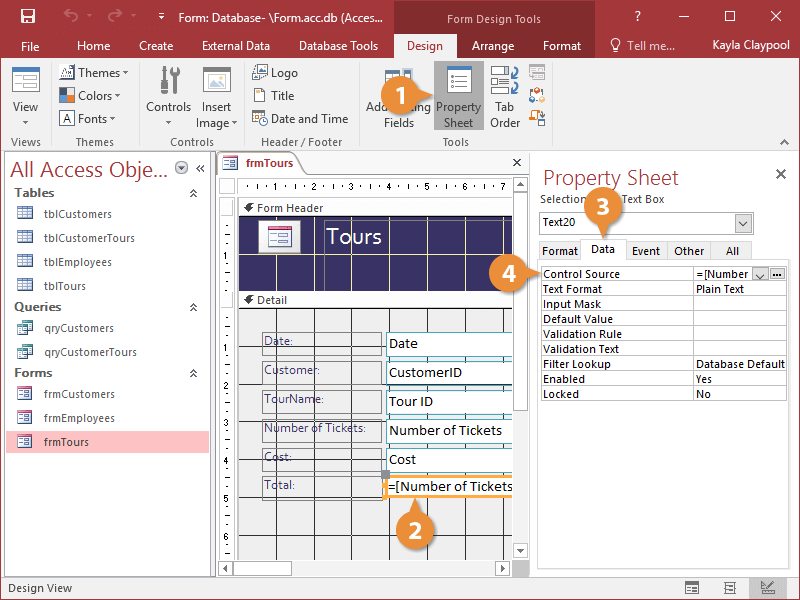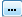Create a Calculated Control | CustomGuide# Create a Calculated Control

How to Create a Calculated Control in AccessA calculated control is an unbound control that displays arithmetic computations on a form. You create calculated controls by entering an expression (or formula) to perform the calculation in the control's Control Source property.

Expressions start with the equal sign (=), which tells Access that you want to perform a calculation.

Next, you must specify the values you want to calculate.

Finally, expressions must contain the arithmetic operators, or function names you want to use to calculate the values.

For example, the formula =[Cost]*[Commission] would multiply the values in the Cost and Commissions fields.

1. Display the form in Design View or Layout View with the Property Sheet displayed.
2. Select the desired control.
3. Click the Data tab.
4. Click the Control Source box and type the expression.### Calculated Control Tips

• You can also enter an expression by clicking thebutton in the Control Source box and using the Expression Builder to create the expression.
• You can also use expressions to create calculated controls and fields in queries and reports
• If a database field name exists in more than one table, you will need to enter the name of the table that contains the field in brackets, followed by an exclamation mark (!). For example, =[tblTours]![Cost]*0.15.